##Maclaurin Chasles-Steiner theorem

Here I expand on Remark-1 of section-2 of Maclaurin.html . There it is mentioned essentially the equivalence of Maclaurin's theorem to that of Chasles-Steiner.
The following property ([ChaslesConiques, p. 72], [PonceletApplications, pp. 308-372], [PonceletTraite, pp. 1-66]) is a generalization of the Maclaurin
Braikenridge theorem.

All N sides XiXi+1 of a polygon p = X1...XN pass through N fixed points Bi. Also all of its vertices but one {X2, ..., XN} say move on fixed lines {a2, ..., aN}.
Then its free vertex X1 describes a conic passing through the two points B1 and B5.
If the points {B1, ..., BN} are collinear, then the conic degenerates to the set consisting of two lines: (a) the line containing points {B1,...,BN} and (b) another line L.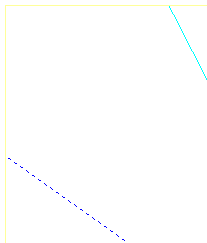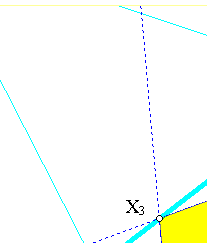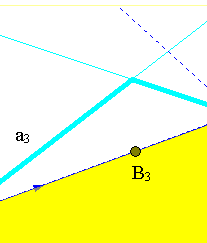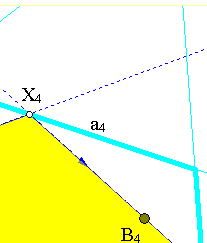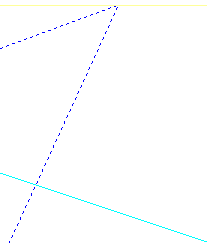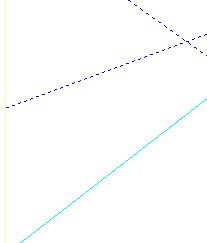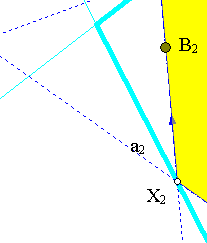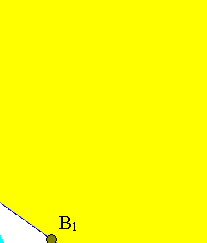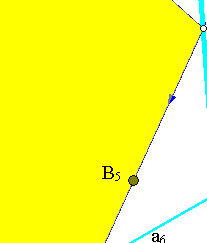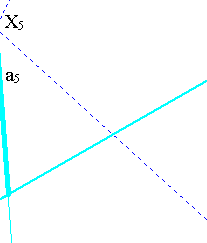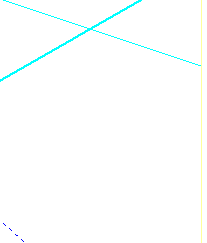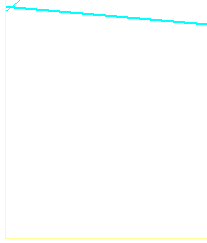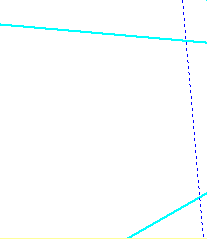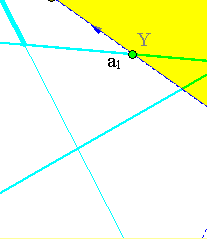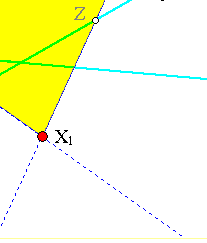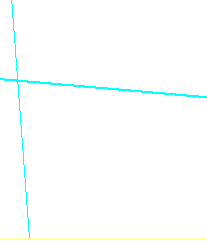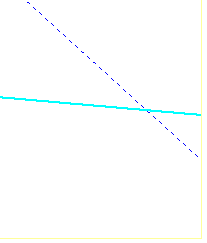The clue here is a combination of the simple fact noticed in RectHypeRelation.html and the Chasles-Steiner theorem ( Chasles_Steiner.html ). To see it draw two
fixed but arbitrary lines {a1, a6} intersecting the first and the last sides of the polygon correspondingly at {Y, Z}. As the polygon changes its position the
following relations are created:
B1: The correspondence Y --> X2 of line a1 to a2 is a perspectivity p1 with center B1.
B2: The correspondence X2 --> X3 of line a2 ot line a3 is a perspectivity p2 with center B2.
....
BN: (N=5 above) The correspondence XN --> Z of line aN to line a6 is a perspectivity pN with center BN.
Thus, the correspondence created by composing all these line-perspectivities is a line-projectivity from line a1 onto line a6:
p = pN * ... * p2 * p1.
Thus, considering the pencils of lines {B*1, B*N} respectively at points {B1, BN} the correspondence of their lines:
B1Y -----> BNZ
is a projective one, hence, by Chasles-Steiner, the intersection-points X1 of these lines two lines will describe a conic passing through {B1, BN}.
The figure below shows an example of such a conic for the case of a variable pentagon.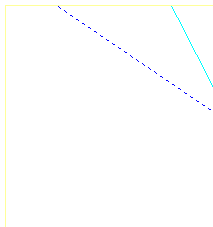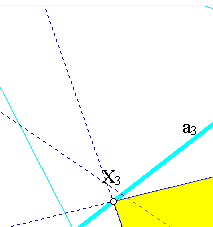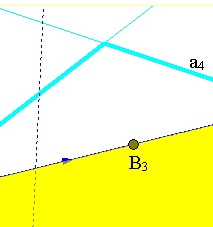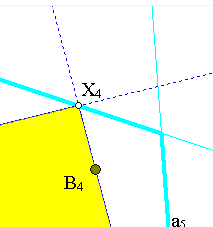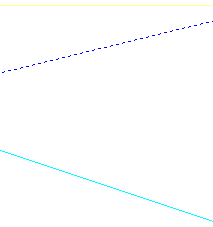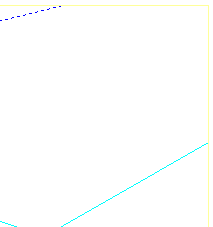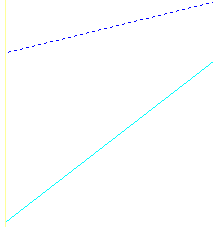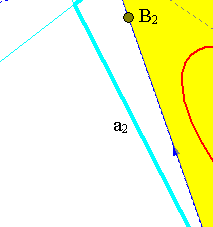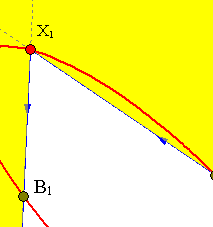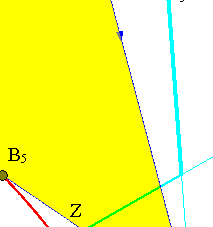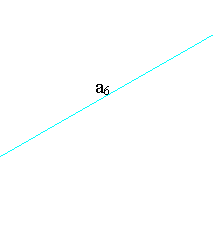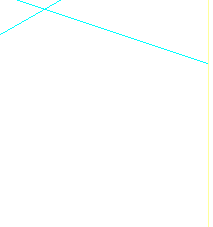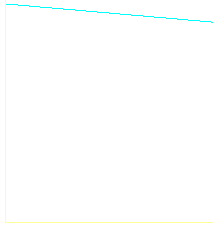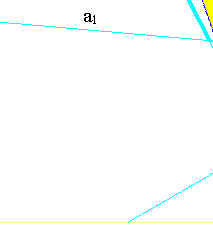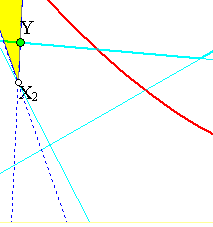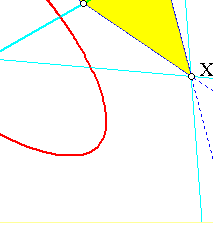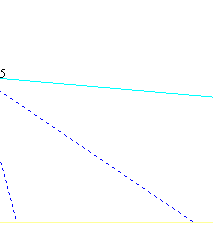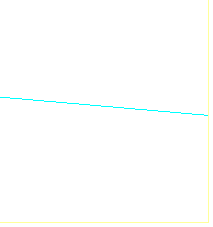The second claim, on the collinearity, follows immediately since in this case a point of the resulting conic is on the line B1BN (containing all other Bi's).
Hence the conic contains the line B1BN and consequently it is degenerate and contains also another line L on which vary the vertices X1 of the polygon.

Remark This particular case of the theorem has an interesting application in the configuration of polygons inscribed into other polygons see for example the file
Polygon_Inscribed_Polygon.html .

### See Also

Maclaurin.html
RectHypeRelation.html
Chasles_Steiner.html
Polygon_Inscribed_Polygon.html

### Bibliography

[ChaslesConiques] Chasles, M. Traite des sections coniques Gauthier-Villars, Paris, 1865
[PonceletApplications] Jean Victor Poncelet Applications d' analyse et de geometrie ... I, II Mallet - Bachellier, Paris 1862
[PonceletTraite] Jean Victor Poncelet Traite de Geometrie Projective I, II Gauthier-Villards, Paris 1865-1866

Return to Gallery

 Produced with EucliDraw© http://users.math.uoc.gr/~pamfilos/